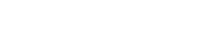## Power from Sunlight – Powering space exploration with solar energy

In this set of activities, students will learn about two concepts that influence solar panel design for space missions: the inverse square law and the angle of incidence.

Students will perform two simple investigations using a photovoltaic cell (solar cell) and a light source.

First, they will measure how the power produced by the solar cells varies with distance from the light source and attempt to retrieve the inverse square law for light intensity experimentally.

Students will then conduct a second experiment to investigate the dependence of the power output for the solar cell with the angle of incidence. Lastly, they will apply these concepts to real ESA space missions.
Subject: Science, Physics
Learning Objectives

• Understanding and calculating light intensity.
• Understanding the angle of incidence.
• Perform hands-on experiments to investigate the inverse square law of light and the impact of the angle of incidence of light.
• Analysing and plotting data.
• Constructing simple electric circuits using solar cells.
• Learning about electric potential difference, electric current, power and light intensity.
• Investigating solar power requirements on space missions.
•
Age range:
14 – 18 years old

Time
Preparation: 1 hour
Experiment Assembly: 20 minutes
Lesson: 1 hour and 30 minutes

Resource available in:
Activity 1: The inverse square law

In this hands-on activity, students will calculate the power output of a solar panel by measuring the electric current and electric potential difference and try to retrieve the inverse square law from their experimental measurements.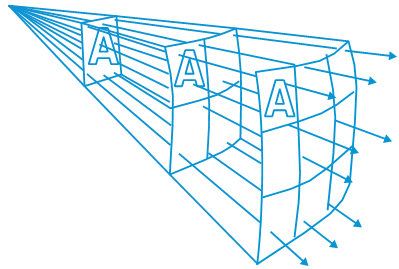Equipment

• Student worksheet printed for each group
• Annex 1 printed for each group
• A dark box (open in one end)
• Pen/pencil
• Electric cables
• Sellotape
• Light source (small light bulb, 4.5V, 0.3A)
• Ruler
• 30 cm rod (for example a wooden stick)
• Material to block light (for example a sponge, cloth)
• Ammeter and voltmeter (or a multimeter)
• Crocodile clips
• Activity 2: The angle of incidence

In this activity, students will learn about the importance of the angle of incidence and the benefits of optimally positioning solar cells. Through an experiment, they will measure how the angle of incidence influences the power output.
Equipment

• Student worksheet printed for each group
• Annex 2 printed for each group
• Pen/pencil
• Experimental setup from Activity 1 (see Annex 2)
• Stick to rotate the solar cell (BBQ stick for example)
• Protractor
• Activity 3: Exploring space with solar power
In this activity, students practice using the inverse square law in application to real ESA space missions. Students will discover how the properties of the inverse square law affect how large the solar panels have to be and how the angle of incidence is of critical importance for missions venturing close to the Sun.
Equipment

• Student worksheet printed for each student
• Pen/pencil
• Calculator
• ### Did you know?

The International Space Station (ISS) is powered by solar panels. The image on the right shows some of the solar panels on the ISS, which is home to up to six astronauts at a time. As the ISS orbits Earth, the solar panels can be rotated to point more directly at the Sun. The panels span an area of 2500 m³ – that is equivalent to the size of half of a football field.

Solar panels on the ISS

Keywords: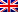EnglishEnglish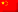Chinese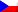Czech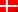Danish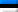Estonian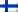Finnish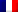French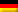German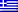Greek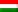Hungarian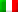Italian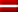Latvian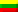Lithuanian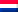Dutch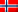Norwegian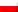Polish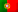Portuguese (Portugal, AO90)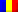Romanian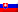Slovak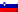Slovenian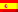Spanish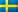Swedish